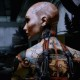# need tutorial/help with 3D applet

topjetboyskypickle shared this question 11 months ago

I am looking for a tutorial on creating an applet in 3D. I copied this one

It is a 3D vector field. To get an idea of what I would like to demonstrate, set the following properties:

p(x,y,z)=-y

q(x,y,z)=-x

r(x,y,z)=-0

This gives the picture of a cylindrical 'tornado' sitting on the xy plane with its vertical axis perpendicular to the xy plane.

I would like to be able to deform the xy plane and maintain the constraint that the z axis of the field remain perpendicular to the xy plane. I would like visualize what happens to this new vector field.1

I think you want p=-y and q=x because "cylindrical"

do you mean something like Sequence(Sequence(Sequence(Vector((k, m, n), (k - 0.5m, m + 0.5k, n)), k, -3, 3, 0.5), m, -3, 3, 0.5), n, 0, 3, 0.5)1

thank you. your code does indeed reproduce the vector field I get with my above inputs. Since I cant wrap my head around the coding in Geogebra, here is what is in the native activity panel

```points = join (list2)

VECTORS=Sequence(Vector(Element(points,i),Element(points,i)+scale (p(Element(points,i)),q(Element(points,i)),r(Element(points,i)))),i,1,(n+1)^(3))

list1=Sequence(Sequence(Sequence((x(A)+ (x(B)-x(A))(i)/(n),y(A)+ (y(B)-y(A))(j)/(n),z(A)+ (z(B)-z(A))(k)/(n)),i,0,n),j,0,n),k,0,n)

list2=Join(list1)
```
Then when you enter the variables in the the three function boxes, it generates the vector field. Anyway, back to my question, how can I deform the xy plane to get a visualization of what the vector field will do with the constraint that the z axis of the vector field is perpendicular to the xy plane.

I guess I need to see a good tutorial on the use of 'sequence'1

I do not understand well you want

can you attach a picture, draw or link for showing an example?1

well, that's why i need help with goegebra, because i cannot draw well what i need to do. Imagine an image you draw on a balloon. Now imagine the distortion of that image as you blow up that balloon.

I want to achieve the same kind of distortion on a vector field.

Look at the attached jpg of a vector field. It is basically a bunch of arrows going around in a circle, parallel to the xy plane. Now imagine curving the xy plane so it approaches the surface of a sphere. I need to depict the change in how the arrows are arranged.